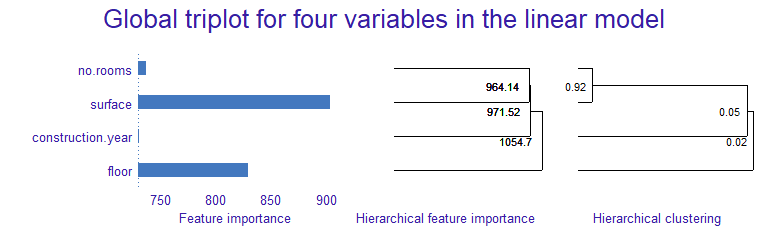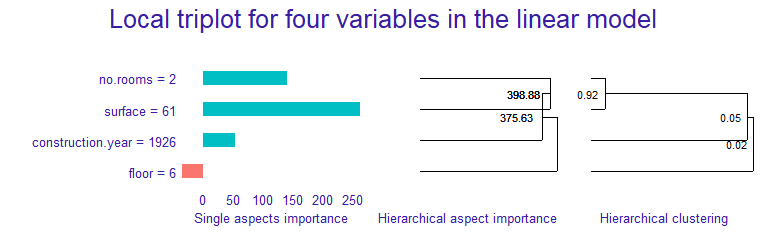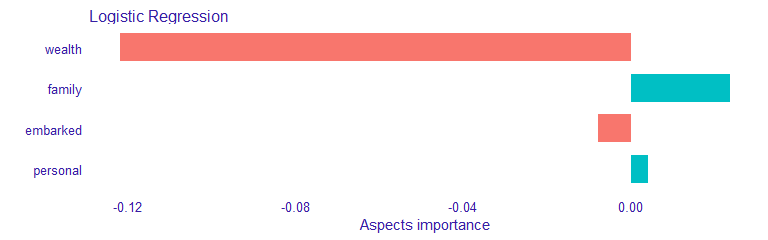## Introduction

The triplot package provides tools for exploration of machine learning predictive models. It contains an instance-level explainer called predict_aspects (AKA aspects_importance), that is able to explain the contribution of the whole groups of explanatory variables. Furthermore, package delivers functionality called triplot - it illustrates how the importance of aspects (group of predictors) change depending on the size of aspects.

Key functions:

• predict_triplot() and model_triplot() for instance- and data-level summary of automatic aspect importance grouping,
• predict_aspects() for calculating the feature groups importance (called aspects importance) for a selected observation,
• group_variables() for grouping of correlated numeric features into aspects.

The triplot package is a part of DrWhy.AI universe. More information about analysis of machine learning models can be found in the Explanatory Model Analysis. Explore, Explain and Examine Predictive Models e-book.## Installation

# from CRAN:
install.packages("triplot")
# from GitHub (development version):
# install.packages("devtools")
devtools::install_github("ModelOriented/triplot")

## Overview

triplot shows, in one place:

• the importance of every single feature,
• hierarchical aspects importance,
• order of grouping features into aspects.

We can use it to investigate the instance level importance of features (using predict_aspects() function) or to illustrate the model level importance of features (using model_parts() function from DALEX package). triplot can be only used on numerical features. More information about this functionality can be found in triplot overview.

### Basic triplot for a model

To showcase triplot, we will choose apartments dataset from DALEX, use it’s numeric features to build a model, create DALEX explainer, use model_triplot() to calculate the triplot object and then plot it with the generic plot() function.

#### Import apartments and train a linear model

library("DALEX")
apartments_num <- apartments[,unlist(lapply(apartments, is.numeric))]

model_apartments <- lm(m2.price ~ ., data = apartments_num)

#### Create an explainer

explain_apartments <- DALEX::explain(model = model_apartments,
data = apartments_num[, -1],
y = apartments_num$m2.price, verbose = FALSE) #### Create a triplot object set.seed(123) library("triplot") tri_apartments <- model_triplot(explain_apartments) plot(tri_apartments) + patchwork::plot_annotation(title = "Global triplot for four variables in the linear model")The left panel shows the global importance of individual variables. Right panel shows global correlation structure visualized by hierarchical clustering. The middle panel shows the importance of groups of variables determined by the hierarchical clustering. At the model level, surface and floor have the biggest contributions. But we also know that Number of rooms and surface are strongly correlated and together have strong influence on the model prediction.Construction year has small influence on the prediction, is not correlated with number of rooms nor surface variables. Adding construction year to them, only slightly increases the importance of this group. ### Basic triplot for an observation Afterwards, we are building triplot for single instance and it’s prediction. (new_apartment <- apartments_num[6, -1]) ## construction.year surface floor no.rooms ## 6 1926 61 6 2 tri_apartments <- predict_triplot(explain_apartments, new_observation = new_apartment) plot(tri_apartments) + patchwork::plot_annotation(title = "Local triplot for four variables in the linear model")The left panel shows the local importance of individual variables (similar to LIME). Right panel shows global correlation structure visualized by hierarchical clustering. The middle panel shows the local importance of groups of variables (similar to LIME) determined by the hierarchical clustering. We can observe that for the given apartment surface has also significant, positive influence on the prediction. Adding number of rooms, increases its contribution. However, adding construction year to those two features, decreases the group importance. We can notice that floor has the small influence on the prediction of this observation, unlike in the model-level analysis. ## Aspect importance for single instance For this example we use titanic dataset with a logistic regression model that predicts passenger survival. Features are combined into thematic aspects. ### Importing dataset and building a logistic regression model set.seed(123) model_titanic_glm <- glm(survived ~ ., titanic_imputed, family = "binomial") ### Manual selection of aspects aspects_titanic <- list( wealth = c("class", "fare"), family = c("sibsp", "parch"), personal = c("age", "gender"), embarked = "embarked" ) ### Select an instance We are interested in explaining the model prediction for the johny_d example. (johny_d <- titanic_imputed[2,]) ## gender age class embarked fare sibsp parch survived ## 2 male 13 3rd Southampton 20.05 0 2 0 predict(model_titanic_glm, johny_d, type = "response") ## 2 ## 0.1531932 It turns out that the model prediction for this passenger’s survival is very low. Let’s see which aspects have the biggest influence on it. We start with DALEX explainer. explain_titanic <- DALEX::explain(model_titanic_glm, data = titanic_imputed, y = titanic_imputed$survived,
label = "Logistic Regression",
verbose = FALSE)

And use it to call triplot::predict_aspects() function. Afterwards, we print and plot function results

library("triplot")

ai_titanic <- predict_aspects(x = explain_titanic,
new_observation = johny_d[,-8],
variable_groups = aspects_titanic)

print(ai_titanic, show_features = TRUE)
##   variable_groups importance     features
## 2          wealth  -0.122049  class, fare
## 3          family   0.023564 sibsp, parch
## 5        embarked  -0.007929     embarked
## 4        personal   0.004069  age, gender
plot(ai_titanic)We can observe that wealth (class, fare) variables have the biggest contribution to the prediction. This contribution is of a negative type. Personal (age, gender) and Family (sibsp, parch) variables have positive influence on the prediction, but it is much smaller. Embarked feature has very small, negative contribution to the prediction.